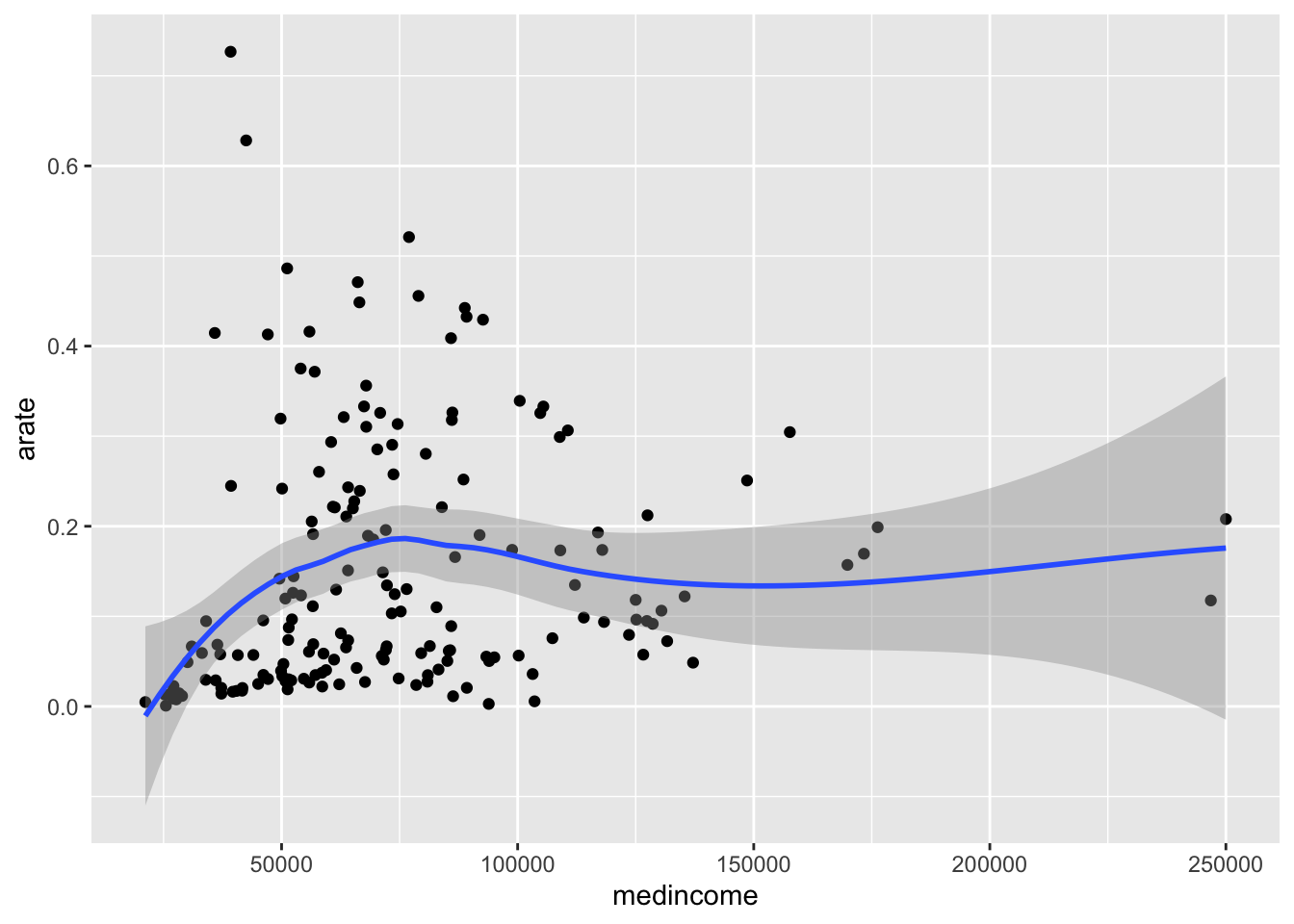2034 字

# 新冠传播中的机会平等

``````library(tidycensus)
library(tidyverse)``````
``## ── Attaching packages ────────────────────────────────── tidyverse 1.3.0 ──``
``````## ✓ ggplot2 3.3.0     ✓ purrr   0.3.3
## ✓ tibble  3.0.0     ✓ dplyr   0.8.5
## ✓ tidyr   1.0.2     ✓ stringr 1.4.0
## ✓ readr   1.3.1     ✓ forcats 0.5.0``````
``````## ── Conflicts ───────────────────────────────────── tidyverse_conflicts() ──
``census_api_key("USE YOUR KEY")``
``## To install your API key for use in future sessions, run this function with `install = TRUE`.``
``````# 纽约市各邮编确诊数据
# 提取2018年人口普查数据
zip <- get_acs(geography = "zcta",
variables = c(medincome = "B19013_001",
population = "B01003_001",
asian = "B02001_005",
black="B02001_003",
white="B02001_002"),
year = 2018)``````
``## Getting data from the 2014-2018 5-year ACS``
``````zipnyc <- merge(covid19nyc,zip,by.x = 'MODZCTA',by.y='GEOID')
# reshape
zipnyc2 <- tidyr::pivot_wider(zipnyc[,-c(3,4,5,8)],names_from = variable, values_from = estimate) %>%
mutate(rate=Positive/population,arate=asian/population,brate=black/population,wrate=white/population)``````

``````# 社区发病率与收入
ggplot(zipnyc2,mapping = aes(medincome,rate))+geom_point()+geom_smooth()``````
``## `geom_smooth()` using method = 'loess' and formula 'y ~ x'``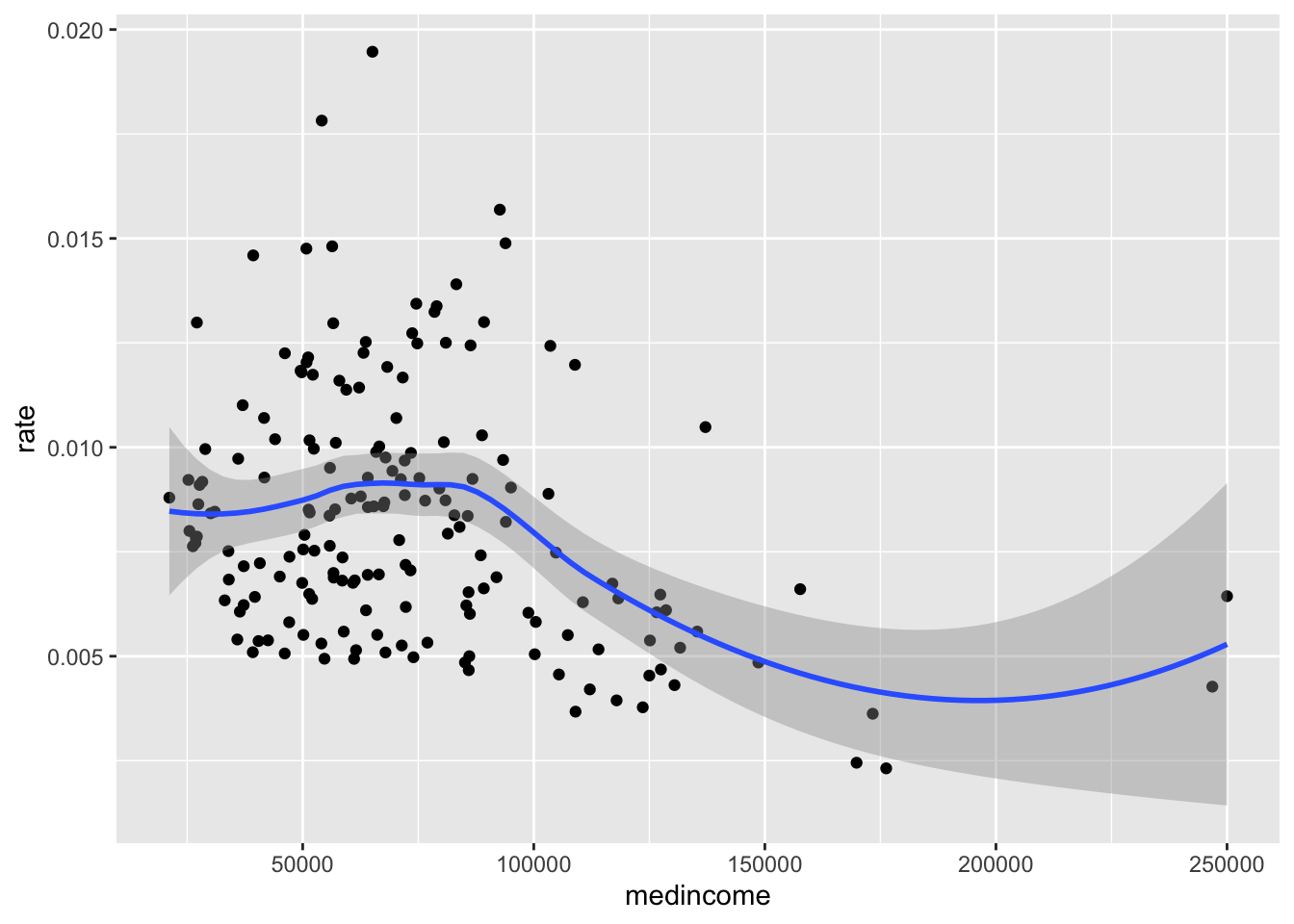``````# 社区发病率与亚裔比例
ggplot(zipnyc2,mapping = aes(arate,rate))+geom_point()+geom_smooth()``````
``## `geom_smooth()` using method = 'loess' and formula 'y ~ x'``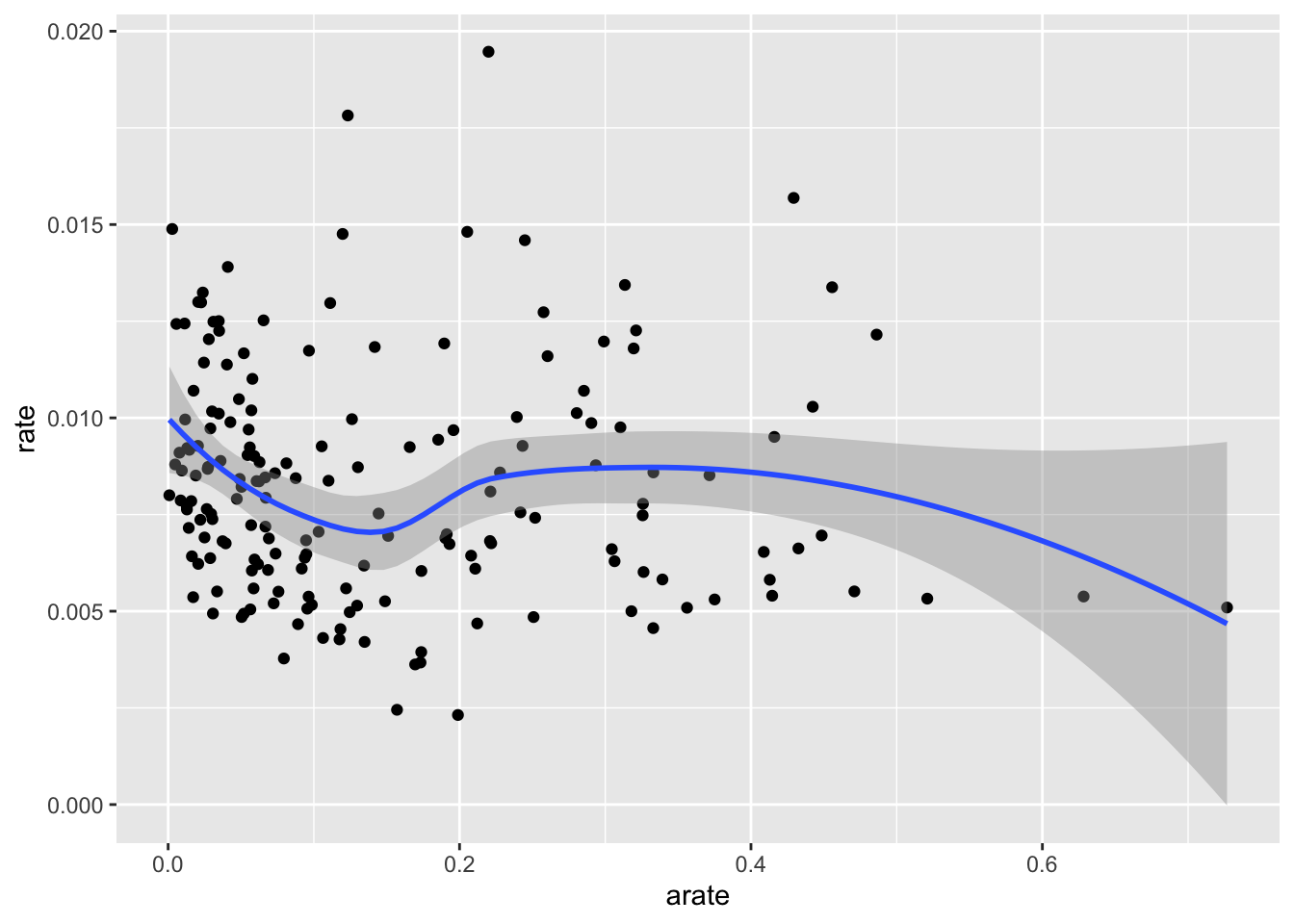``````# 社区发病率与非裔比例
ggplot(zipnyc2,mapping = aes(brate,rate))+geom_point()+geom_smooth()``````
``## `geom_smooth()` using method = 'loess' and formula 'y ~ x'``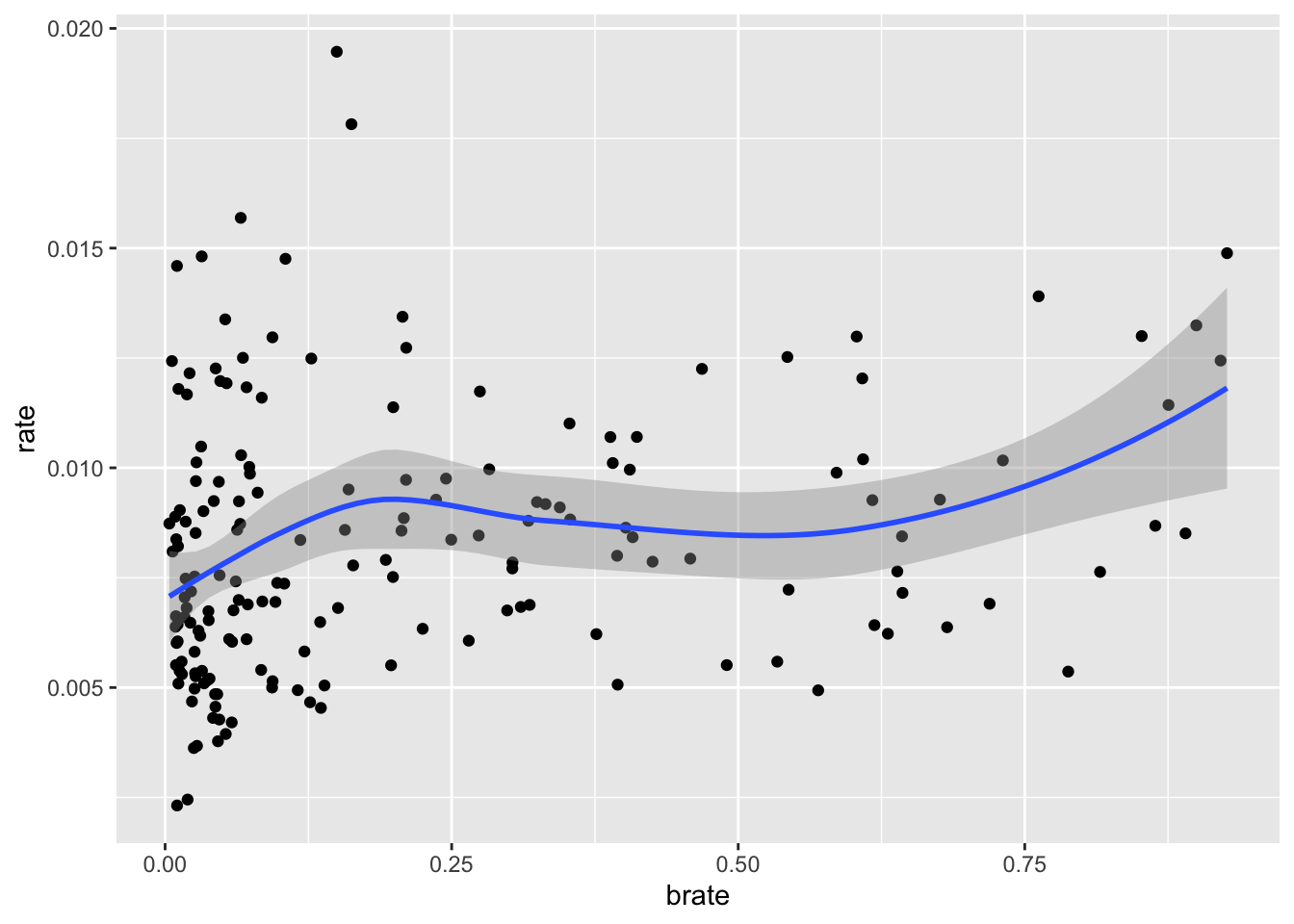``````# 社区发病率与白人比例
ggplot(zipnyc2,mapping = aes(wrate,rate))+geom_point()+geom_smooth()``````
``## `geom_smooth()` using method = 'loess' and formula 'y ~ x'``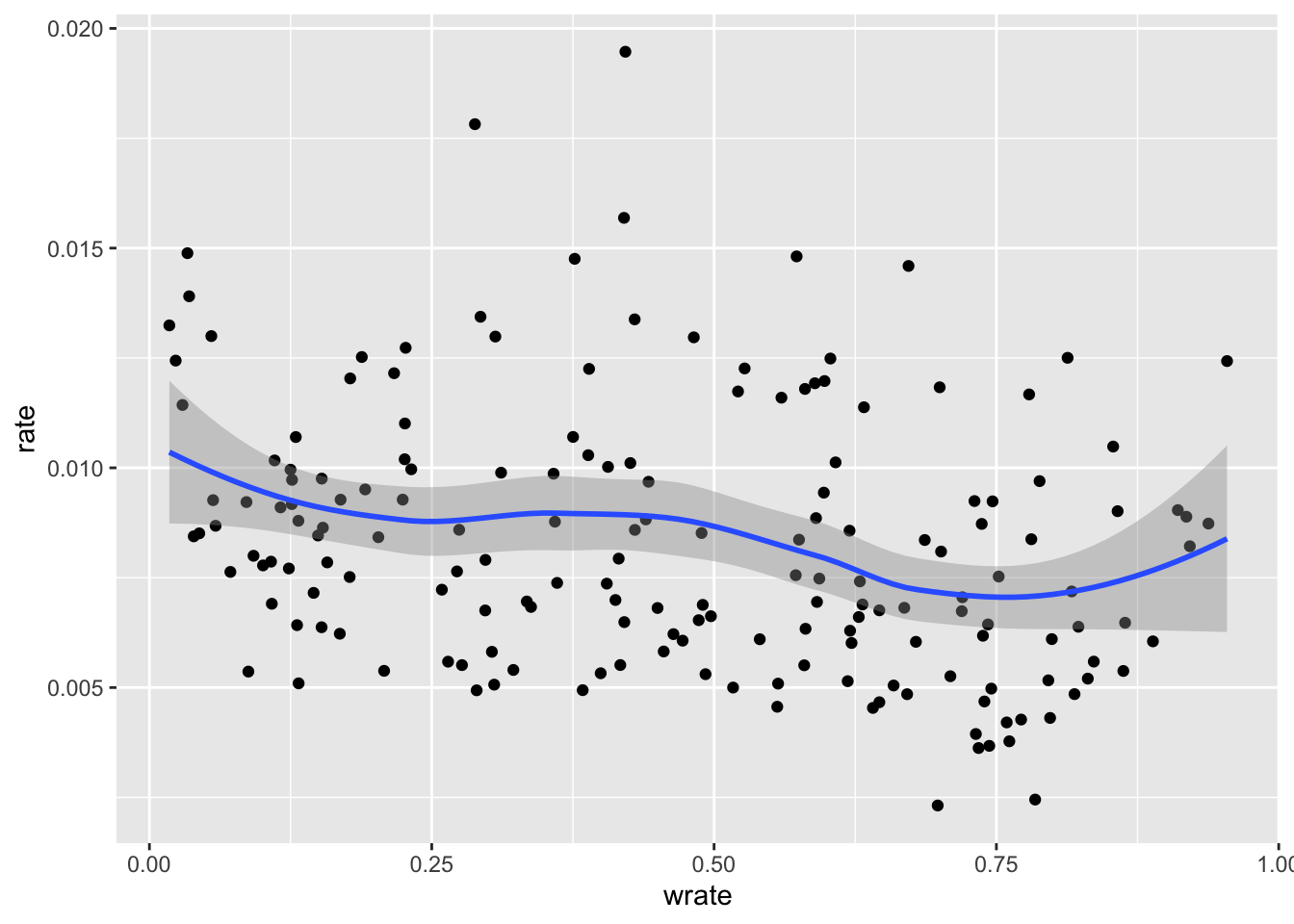``````# 白人比例与收入
ggplot(zipnyc2,mapping = aes(medincome,wrate))+geom_point()+geom_smooth()``````
``## `geom_smooth()` using method = 'loess' and formula 'y ~ x'``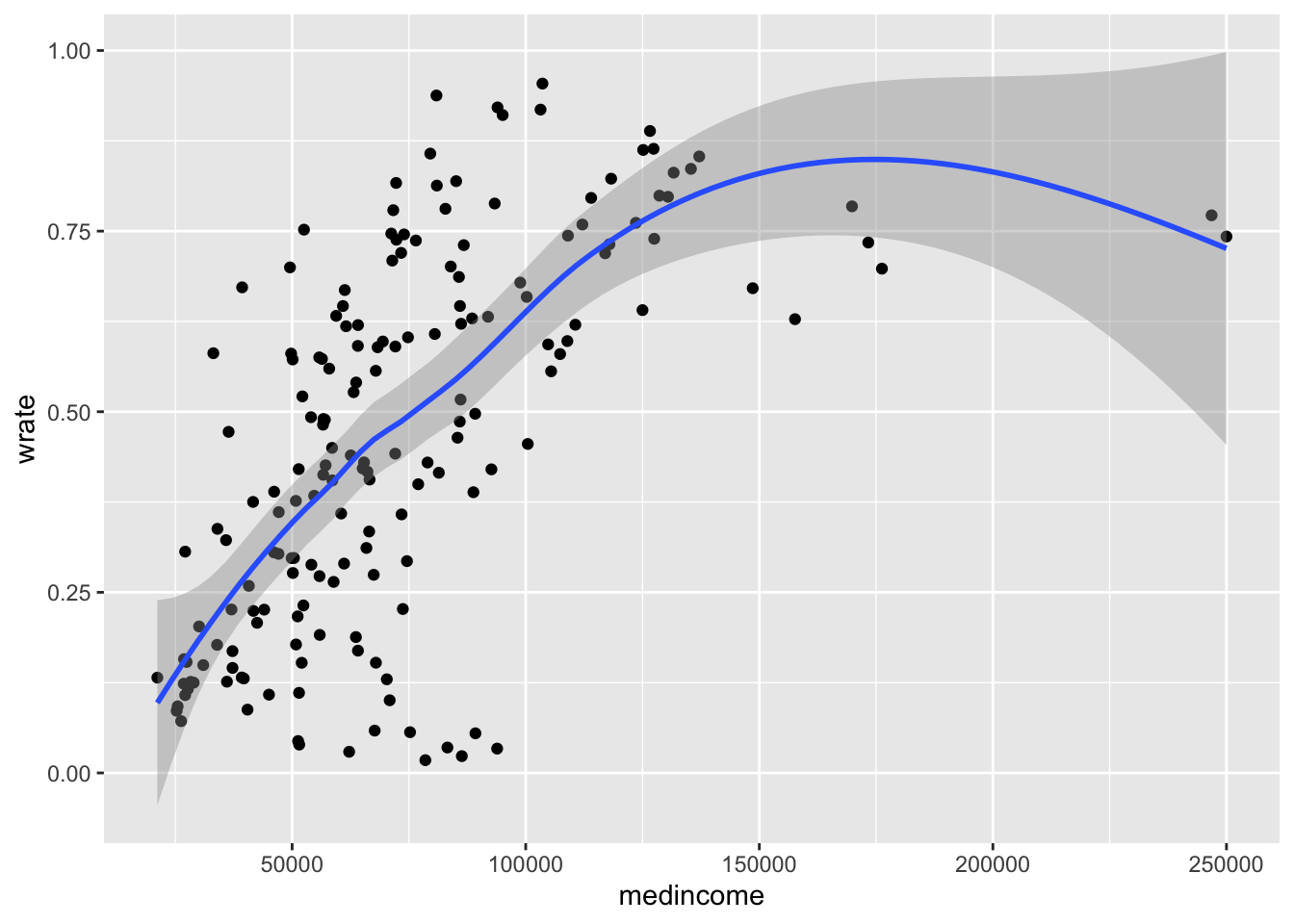``````# 黑人比例与收入
ggplot(zipnyc2,mapping = aes(medincome,brate))+geom_point()+geom_smooth()``````
``## `geom_smooth()` using method = 'loess' and formula 'y ~ x'````````# 亚裔比例与收入
ggplot(zipnyc2,mapping = aes(medincome,arate))+geom_point()+geom_smooth()``````
``## `geom_smooth()` using method = 'loess' and formula 'y ~ x'``